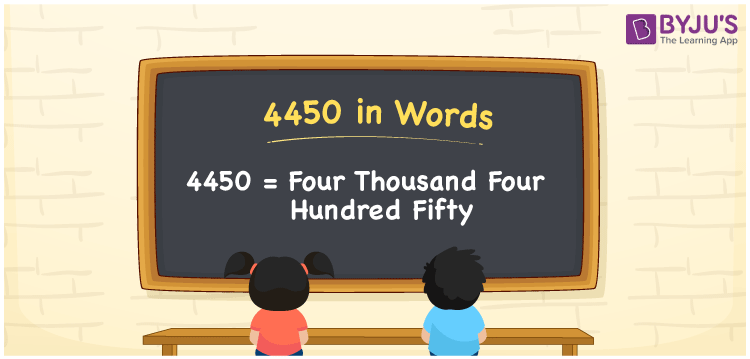# 4450 in Words

4450 in words is written as Four thousand four hundred fifty. In both the International System of Numerals and the Indian System of Numerals, 4450 is written as Four thousand four hundred fifty. The number 4450 is a Cardinal Number as it could represent some quantity. For example, “this video got 4450 likes today”.

 4450 in Words Four thousand four hundred fifty Four thousand four hundred fifty in Number 4450

## 4450 in English Words

4450 in English words is read as “Four thousand four hundred fifty”.## How to Write 4450 in Words?

To write 4450 in words, we shall use the place value chart. In the place value chart, put 4 in the thousands, 4 in the hundreds, 5 in the tens, and 0 in the ones, respectively. Let us make a place value chart to write the number 4450 in words.

 Thousands Hundreds Tens Ones 4 4 5 0

Thus, we can write the expanded form as

4 × Thousand + 4 × Hundred + 5 × Ten + 0 × One

= 4 × 1000 + 4 × 100 + 5 × 10 + 0 × 1

= 4000 + 400 + 50 + 0

= 4450

= Four thousand four hundred fifty.

4450 is a natural number which is the successor of 4449 and the predecessor of 4451.

4450 in words – Four thousand four hundred fifty

• Is 4450 an odd number? – No
• Is 4450 an even number? – Yes
• Is 4450 a perfect square number? – No
• Is 4450 a perfect cube number? – No
• Is 4450 a prime number? – No
• Is 4450 a composite number? – Yes

## Frequently Asked Questions on 4450 in Words

Q1

### How to write 4450 in words?

4450 in words is written as Four thousand four hundred fifty.
Q2

### How to write 4450 in the International and Indian System of Numerals?

In both, the system of numerals, 4450 in words, is written as Four thousand four hundred fifty.
Q3

### How to write 4450 in a place value chart?

In the place value chart, write 4 in the thousands, 4 in the hundreds, 5 in the tens, and 0 in the ones, respectively.